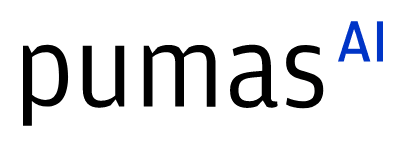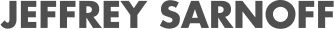07/26/2023, 8:30 PM9:00 PM UTC
32-D463 (Star)

#### Abstract:

Automatic differentiation (AD) is great: use gradients to optimize, sample faster, or just for fun! But what about coin flips? Agent-based models? Nope, these aren’t differentiable... or are they? StochasticAD.jl is an open-source research package for AD of stochastic programs, implementing AD algorithms for handling programs that can contain discrete randomness.

#### Description:

StochasticAD.jl is an open-source research package for automatic differentiation (AD) of stochastic programs. The particular focus is on implementing AD algorithms for handling programs that can contain discrete randomness. But what does this even mean?

Derivatives are all about how functions are affected by a tiny change ε in their input. For example, take the function sin(x). Perturb x by ε, and the output changes by approximately cos(x) * ε: tiny change in, tiny change out. And the coefficient cos(x)? That's the derivative!

But what happens if your function is discrete and random? For example, take a Bernoulli variable, with probability p of being 1 and probability 1-p of being 0. If we perturb p by ε, the output of the Bernoulli variable cannot change by a tiny amount. But in the probabilistic world, there is another way to change by a tiny amount on average: jump by a large amount, with tiny probability.

StochasticAD.jl generalizes the well-known concept of dual numbers by including a third component to describe large perturbations with infinitesimal probability. The resulting object is called a stochastic triple, and StochasticAD.jl develops the algorithms to propagate this triple through user-written code involving discrete randomness. Ultimately, the result is a provably unbiased estimate of the derivative of your program, even if it contains discrete randomness!

In this talk, we will discuss the workings of StochasticAD.jl, including the underlying theory and the technical implementation challenges.